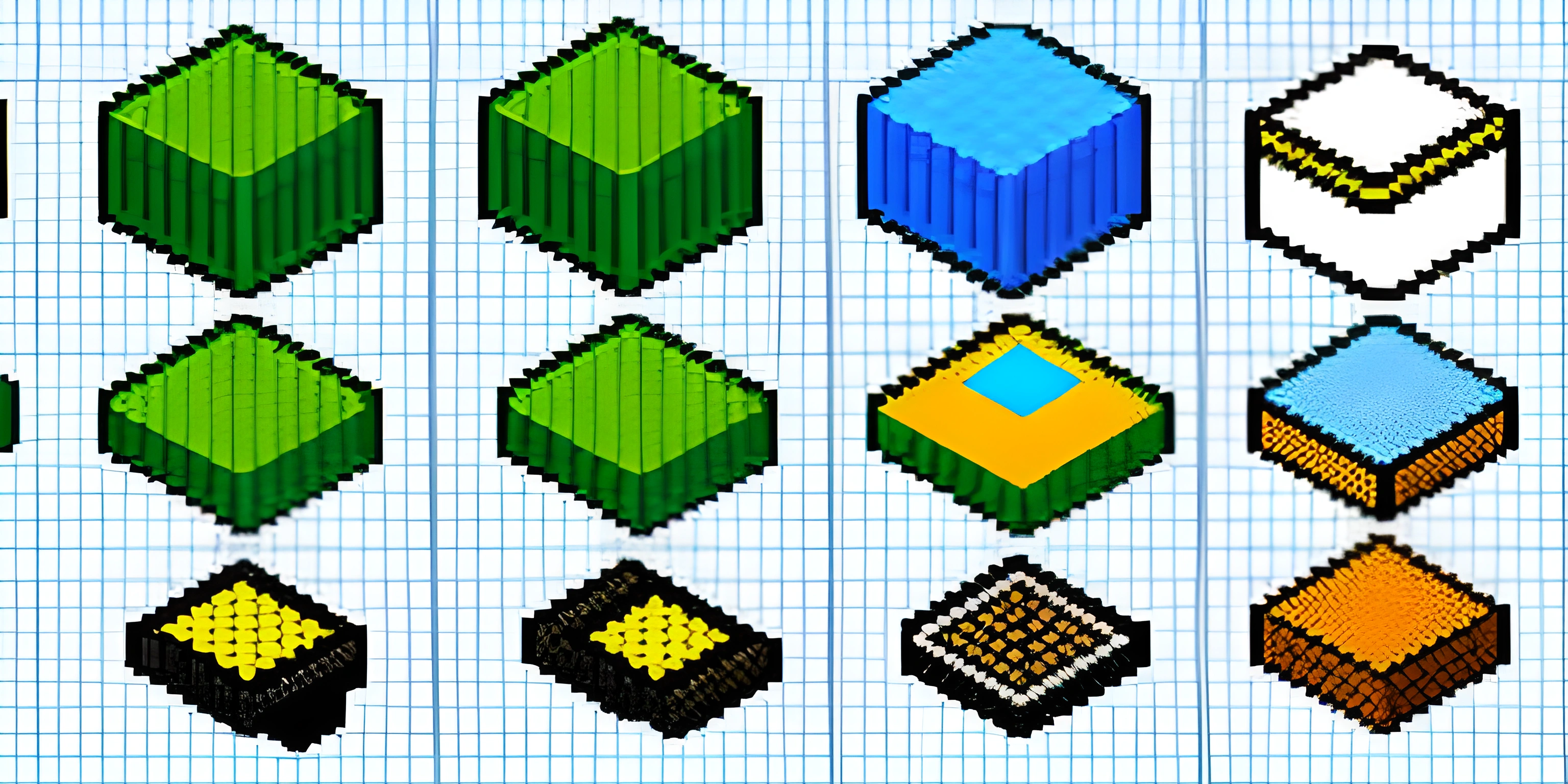# Working with Arrays and Objects in JavaScriptNote: this page has been created with the use of AI. Please take caution, and note that the content of this page does not necessarily reflect the opinion of Cratecode.

JavaScript is a versatile programming language that allows you to work with various data structures. Two of the most commonly used data structures in JavaScript are arrays and objects. In this article, we will explore how to create, manipulate, and use arrays and objects in JavaScript.

## Arrays

Arrays are ordered collections of elements, which can be of any data type, including other arrays and objects. They are created using square brackets `[]`.

### Creating Arrays

Here's how to create an array:

``let myArray = [1, "two", 3, "four"];``

### Accessing Array Elements

To access an array element, use its index (zero-based) within square brackets:

``````let firstElement = myArray; // 1
let secondElement = myArray; // "two"``````

### Modifying Array Elements

To modify an array element, assign a new value to the desired index:

``myArray = "three";``

### Array Methods

JavaScript provides several built-in methods for manipulating arrays, such as `push()`, `pop()`, `shift()`, `unshift()`, `splice()`, and more.

## Objects

Objects are collections of key-value pairs, where keys are strings, and values can be of any data type, including other objects and arrays. They are created using curly braces `{}`.

### Creating Objects

Here's how to create an object:

``````let myObject = {
key1: "value1",
key2: 2,
key3: ["value3a", "value3b"]
};``````

### Accessing Object Properties

To access an object property, use either dot notation or square brackets with the property name:

``````let value1 = myObject.key1; // "value1"
let value2 = myObject["key2"]; // 2``````

### Modifying Object Properties

To modify an object property, assign a new value to the desired key:

``myObject.key1 = "newValue1";``

### Object Methods

JavaScript provides several built-in methods for manipulating objects, such as `Object.keys()`, `Object.values()`, `Object.entries()`, and more.

## Conclusion

Arrays and objects are essential data structures in JavaScript, allowing you to store and manipulate various types of data. By understanding how to create, access, and modify arrays and objects, you can build more complex and dynamic applications.

## FAQ

### What are the differences between arrays and objects in JavaScript?

In JavaScript, arrays and objects are both used to store data, but they have different structures and purposes. Arrays are ordered collections of elements, where each element has an index, starting from 0. Objects, on the other hand, are unordered collections of key-value pairs, where each key is a string or a symbol, and each value can be any data type.

### How do I create and access elements in a JavaScript array?

To create an array in JavaScript, you can either use the `Array` constructor or the array literal syntax. Here's an example:

``````// Using the Array constructor
let myArray = new Array(1, 2, 3);
// Using the array literal syntax
let myArray = [1, 2, 3];``````

To access elements in an array, use the index of the element you want to access, like so:

``````let firstElement = myArray; // 1
let secondElement = myArray; // 2
let thirdElement = myArray; // 3``````

### How do I create and access properties in a JavaScript object?

To create an object in JavaScript, you can either use the `Object` constructor or the object literal syntax. Here's an example:

``````// Using the Object constructor
let myObject = new Object();
myObject.name = "Alice";
myObject.age = 30;
// Using the object literal syntax
let myObject = {
name: "Alice",
age: 30
};``````

To access properties in an object, you can use either the dot notation or the bracket notation, like so:

``````let name = myObject.name; // "Alice"
let age = myObject["age"]; // 30``````

### Can I combine arrays and objects in JavaScript?

Yes, you can combine arrays and objects in JavaScript to create more complex data structures. For example, you can have an array of objects or an object with properties that are arrays. Here's an example:

``````let employees = [
{ name: "Alice", age: 30, role: "developer" },
{ name: "Bob", age: 28, role: "designer" },
{ name: "Charlie", age: 25, role: "manager" }
];
let company = {
name: "Cratecode",
employees: [
{ name: "Alice", age: 30, role: "developer" },
{ name: "Bob", age: 28, role: "designer" },
{ name: "Charlie", age: 25, role: "manager" }
]
};``````

### How do I add, remove, or modify elements in arrays and objects?

To add, remove, or modify elements in arrays, you can use various built-in methods like `push`, `pop`, `shift`, `unshift`, `splice`, and more. For objects, you can simply assign a new value to an existing property, add a new property, or delete a property using the `delete` keyword. Here are some examples:

``````// Add an element to an array
myArray.push(4); // [1, 2, 3, 4]
// Remove an element from an array
myArray.pop(); // [1, 2, 3]
// Modify an element in an array
myArray = 99; // [1, 99, 3]
// Add or modify a property in an object
myObject.gender = "female"; // { name: "Alice", age: 30, gender: "female" }
// Remove a property from an object
delete myObject.age; // { name: "Alice", gender: "female" }``````